### Schedule

#### 2015-04-23

TimeSpeaker/ Title/ Abstract/ VODLocation
09:50 - 10:00 개회사
CAMP 대강당
10:00 - 11:00 멘토 강연 1
Jang Soo Kim (Sungkyunkwan University),
1. Topic : Project on combinatorics of orthogonal polynomials Enumeration of objects is a powerful tool for understanding their properties, and revealing hidden connections between them. Among other things, constructing explicit bijections is widely considered to be the best way to enumerate objects because bijections give us insight to understand how objects are related to each other. An interesting feature of combinatorics is that it is often related to other fields in mathematics. In this research project we will focus on the combinatorics of orthogonal polynomials. Orthogonal polynomials are a family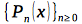of polynomials satisfying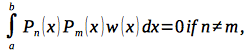for constants a, b and a weight function w(x) . The quantity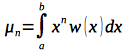is called the nth moment. It is well known that any monic orthogonal polynomials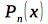satisfy a three-term recurrence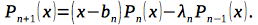Viennot  showed that the moment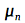is the sum of weights of weighted Motzkin paths of length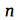whose weights are defined using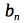and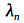. Viennot's theory provides us a connection between orthogonal polynomials and combinatorics. Problem 1. Find a combinatorial proof of the following identities in  :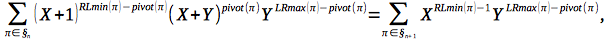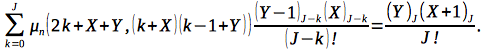Problem 2. Prove the orthogonality of associate Laguerre polynomials in  combinatorially. Find the linearization coefficients. Problem 3. Prove the conjecture of Drake  on a formula for the linearization coefficients of associate Hermite polynomials. Problem 4. Find a combinatorial proof of the linearlization formula for q-Laguerre polynomials due to Kasraoui, Stanton, Zeng . -References-  Dan Drake.The combinatorics of associated Hermite polynomials. European J. Combin., 30(4):1005--1021, 2009.  Anisse Kasraoui, Dennis Stanton, and Jiang Zeng. The combinatorics of Al-Salam-Chihara q-Laguerre polynomials. Adv. in Appl. Math., 47(2):216--239, 2011.  Dongsu Kim, Dennis Stanton, and Jiang Zeng. The combinatorics of the Al-Salam-Chihara q-Charlier polynomials. Se ́m. Lothar. Combin., 54:Art. B54i, 15 pp. (electronic), 2005/07.  Jang~Soo Kim and Dennis Stanton. The combinatorics of associated Laguerre polynomials, http://arxiv.org/abs/1501.03880}.  Ge ́rard Viennot. A combinatorial theory for general orthogonal polynomials with extensions and applications. Orthogonal polynomials and applications (Bar-le-Duc,1984)}, volume 1171 of Lecture Notes in Math., pages 139--157. Springer, Berlin, 1985. 2. Prerequisites The references above. 3. The optimum number of students 4 students who are interested in combinatorics
CAMP 대강당
11:00 - 11:30 휴식 및 토론
11:30 - 12:30 멘토 강연 2
Jeaman Ahn (Kongju National University),
1. Topic : Gröbner Bases Theory and Applications in Algebraic Geometry The algorithmic theory of Gröbner bases has been introduced, in 1965, by Bruno Buchberger with various forerunners since the end of the 19th century and various related theories. In the meantime, the method of Gröbner bases has been heavily studied and is now available in all major mathematical software systems. It has found numerous applications in abstract fields of mathematics as well as in science and engineering. In this lecture, we give a brief overview on Gröbner bases theory, addressed to novices without prior knowledge in the field. After explaining the concept of Gröbner bases by studying uniquenss of polynomial division, we apply Gröbner bases for computing examples from polynomial equation solving and algebraic relations. For non-experts with modest background, a short discussion of complexity issues will be provided with some historical remarks. -References-  D. Cox, J. Little, D. O’Shea, Ideals, Varieties and Algorithms, Third Edition, Undergraduate, Springer, New York-Berlin-Heidelberg.  Mike Stillman, Gröbner Bases: a Tutorial,  D. Eisenbud (1995): Commutative Algebra with an eye towards Algebraic Geometry (Chap 15), Springer, New York-Berlin-Heidelberg.  Bayer, Dave; Mumford, David, What can be computed in algebraic geometry? Computational algebraic geometry and commutative algebra (Cortona, 1991), 1–48, Sympos. Math., XXXIV, Cambridge Univ. Press, Cambridge, 1993. 2. Prerequisites Algebra(graduate course), commutative algebra, algebraic geometry 3. The optimum number of students 4~6 students in each team
CAMP 대강당
12:30 - 13:30 점심
13:30 - 14:30 멘토 강연 3
Youngho Woo (NIMS),
1. Topic : Geometry of tensors and its application For detail information about this topic, visit the website http://wiki.siam.org/siag-ag/index.php/SIAM_AG_15_Proposed_Minisymposia The related topics in Minisymposia (in SIAM AG15) are i) Spectral Theory of Tensors and Tensor Rank, ii) Group actions in algebraic geometry and commutative algebra, iii) Tensor decomposition: ideals meet applications, iv) Geometry of Matrix Multiplication. The main topic of the lecture is a computation of tensor rank focused on cases of symmetric tensors or equivalently homogeneous polynomials. Tensor rank is a natural generalization of rank of matrices, which is a fundamental concept in basic linear algebra. One may think of a tensor as a multidimensional array of numbers. Defining "rank", however, is not obvious mainly because a several equivalent definitions of rank of 2-dimesional array (= matrix) are not equivalent to each other in a general tensor anymore! The first part of the lecture will consist of defining rank of a tensor and comparison to the 2-dimensional case. In the second part, we will focus on symmetric tensors. If we think of a symmetric tensor as a homogeneous polynomial ( = a form), then rank ( symmetric rank ) of a form is called "Waring rank" sometimes because there is a similar thing, "Waring problem" in number theory. The symmetric case has a long history. J. J. Sylvester's result on a binary form in Victorian age is influential even today and its generalization to multi-variables is settled recently. His original idea is formulated in a modern algebraic geometry language, so called "Apolarity lemma" which is still a main technique to compute the rank of a given form. We will give some examples whose rank are computed using Apolarity lemma. For generic tensors or forms, rank is closely related to dimension of various secant varieties of classical projective varieties, for example, Veronese variety or Segre variety. To find equations of these secant varieties is one of main topics in applied side. -References-  [J.M. Landsberg] Tensors : Geometry and applications.  [E. Carlini, N. Grieve, L. Oeding] Four lectures on secant varieties. arXiv:1309.4145v1 2. Prerequisites Linear algebra (undergraduate level), familiarity with basic concepts of algebraic geometry (e.g. projective spaces, algebraic varieties, defining ideals, etc.) 3. The optimum number of students 4~6
CAMP 대강당
14:30 - 15:30 멘토 강연 4
Kwankyu Lee (Chosun University),
1. Topic : Introduction to algebraic geometric coding theory and its further studies 이 모임에서는 대수기하 부호에 관한 간단한 이해를 하고 좀 더 심층적인 연구를 원하는 사람은 최근 본인이 관심을 갖고 있는 Ordinary and Relative Generalized Hamming Weights of Algebraic Geometry에 대하여 연구하도록 한다.

(Ordinary and Relative Generalized Hamming Weights of Algebraic Geometry)

Recently a good bound for the generalized Hamming weights of multi-point evaluation and differential AG codes was proposed in . It is a natural generalization of the well-known order bound for one-point AG codes. This bound can be used to bound or, if we are lucky, determine some higher generalized Hamming weight of multi-point AG codes. In , by a slight generalization, we also obtain a bound for the relative generalized Hamming weights, which are essential measures of the performance of secret sharing schemes based on linear codes. This provides information on the access structure of secret sharing schemes based on AG codes and its subcodes. Upon this recent achievement, we can think of two further research projects. First one, which seems hard and may be beyond the scope of uninitiated students and even the author of  himself, is to widen the applicability of the results in  by removing an important requirement on the AG codes to which the bound is applied. Second one, which is quite straightforward and computational, is to find more examples in which the bounds in  can be successfully used. -References-  H. Stichtenoth, Algebraic Function Fields and Codes, 2nd Edition, Springer-Verlag, 2009.  M. Tsfasman, S. Vladut, Geometric approach to higher weights, IEEE Trans. Inf. Theory 41 (6) (1995) 1564–1588.  K. Lee, Bounds for generalized Hamming weights of general AG Codes, Preprint. 2. Prerequisites Coding theory, algebraic curve, MAGMA 3. The optimum number of students Not more than 4 students
CAMP 대강당
15:30 - 16:30 휴식 및 토론
16:30 - 17:30 멘토 강연 5
Soojoon Lee (Kyung Hee University),
1. Topic : 숨은 부분군 문제에 대한 양자 알고리즘

(Quantum algorithms for the hidden subgroup problem)

양자 계산은 지수적으로 많은 입력 데이터를 균등한 확률 분포를 갖는 하나의 중첩된 상태로 표현하여, 특정한 함수를 통해서 모든 입력에 대한 출력을 병렬로 한 번에 처리할 수 있다. 이것을 양자 병렬계산이라고 하는데 이를 통하여 기존의 계산에서 할 수 없었던 놀라운 계산 능력을 보여주었다. 특히, 1994년에 Peter W. Shor가 양자 Fourier 변환을 이용하여 소인수 분해 문제를 다항시간에 해결하는 양자 소인수 분해 알고리즘을 개발함으로써, 양자 계산이 현재 주로 사용되고 있는 몇 가지 공개키 암호 체계에 큰 영향을 줄 수 있다는 사실이 알려지게 되었다. 한편, G를 주어진 finite group이라고 하고, H를 그 group G의 임의의 한 subgroup 이라고 하자. 함수 f가 다음과 같은 성질,

f(a)=f(b) <=> aH=bH,

를 만족하는 G에서 정의된 임의의 함수라고 할 때, 함수 f를 이용하여 subgroup H를 찾는 문제를 hidden subgroup problem(HSP)이라고 한다. 흥미롭게도 양자 소인수 분해 알고리즘은 abelian group에서의 HSP를 해결하는 양자 알고리즘으로 일반화될 수 있고, graph isomorphism problem과 어떤 lattice problem은 각각 특정한 non-abelian group에서의 HSP와 관련이 되기 때문에, 일반적인 group에서의 HSP를 해결하는 것이 양자 계산 이론에서도 기존의 계산 이론에서도 중요한 문제로 여겨졌다. 여기에서 가장 단순한 형태의 non-abelian group 중의 하나인 두 cyclic group의 semi-direct product group위에서의 HSP를 고려하고, 이미 알려져 있는 결과를 이해하여 이를 확장하고자 한다. -References-  Michael A. Nielsen and Isaac L. Chuang, "Quantum Computation and Quantum Information", Cambridge University Press, 2010 (Chap 5. 참고, 특히 Sec. 5.4)  D. P. Chi, J. S. Kim, and S. Lee, "Quantum algorithms for the hidden subgroup problem on some semi-direct product groups by reduction to Abelian cases", Physics Letters A 359 (2006) 114–116. 2. Prerequisites Group Theory 3. The optimum number of students 4~6
CAMP 대강당
17:30 - 18:30 멘토 강연 6
Donghoon Hyeon (Seoul National University),
1. Topic : Toric geometry and computation Toric varieties are arguably the most accessible (and fun!) part in modern algebraic geometry where most if not all things can be concretely computed. It has a beautiful interplay between convex geometry and computational commutative algebra. I will cover basic elements of these different fields and explain how they all work together in the study of toric geometry. -References-  Toric varieties, by David A. Cox, John B. Little, and Henry K. Schenck  Groebner bases and convex polytopes, by B. Sturmfels 2. Prerequisites A first course knowledge in commutative algebra and algebraic geometry 3. The optimum number of students 4~6
CAMP 대강당
18:30 - 20:00 저녁 식사
20:00 - 22:00 토론 및 발표 준비
호텔 내

#### 2015-04-24

TimeSpeaker/ Title/ Abstract/ VODLocation
09:30 - 10:30 질문 및 토론
10:30 - 11:30 학생팀 발표 1
CAMP 대강당
11:30 - 12:30 학생팀 발표 2
CAMP 대강당
12:30 - 13:30 점심
13:30 - 14:30 학생팀 발표 3
CAMP 대강당
14:30 - 15:30 학생팀 발표 4
CAMP 대강당
15:30 - 16:00 휴식 및 토론
16:00 - 17:00 학생팀 발표 5
CAMP 대강당
17:00 - 18:00 학생팀 발표 6
CAMP 대강당
18:00 - 19:30 식사 및 귀가